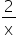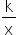Mathematics
Easy

Question

# What are the asymptotes of the function f(x) = -?

## x = -2 , y = 0x = 2 , y = -2x = 0 , y = 0x = 0 , y = -2Hint:

## The correct answer is: x = 0 , y = 0

### Here, the function is in the form: y =.Comparing the equation with y = -2/x, we geth =0 and k =0.Thus, Vertical asymptote: x = 0 Horizontal asymptote: y = 0.Hence, the correct option is A.

Vertical asymptote is x =0 and horizontal asymptote is y = 0.

### Related Questions to study#### With Turito Foundation.#### Get an Expert Advice From Turito.